# The Snub 24-cell

The snub 24-cell is a uniform polychoron that can be used as an intermediate to derive the 600-cell from the 24-cell. It is bounded by 24 icosahedra, corresponding with the cells of a 24-cell, and 120 tetrahedra filling in the gaps between them, for a total of 144 cells, 480 triangles, 432 edges, and 96 vertices.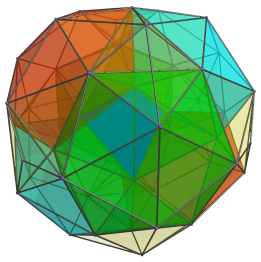## Structure

We shall explore the structure of the snub 24-cell by means of its parallel projection into 3D, centered on an icosahedral cell.

### Northern Hemisphere

We start with the nearest icosahedral cell in the “northern hemisphere” of the snub 24-cell.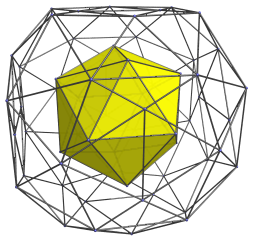For clarity, only the skeleton of the projection envelope is shown in addition to the nearest cell. Twelve of the icosahedron's faces are shared with tetrahedral cells, as shown in the next image: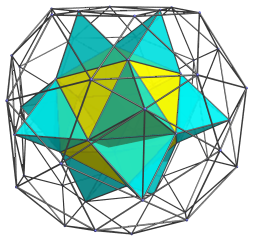These tetrahedra are part of a set of 96 tetrahedra that share a face with an icosahedron. There is another set of 24 tetrahedra that share faces only with tetrahedra in the first set. Six of these latter tetrahedra are shown below: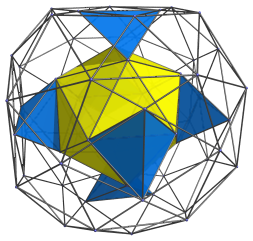As can be seen, these tetrahedra share an edge with the nearest icosahedron, and straddle each neighbouring pair of cells from the previous image. The next image shows both groups of cells together: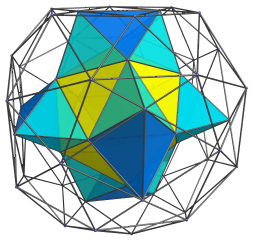The remaining eight faces of the nearest icosahedron are joined to 8 other icosahedra, 4 of which are shown next: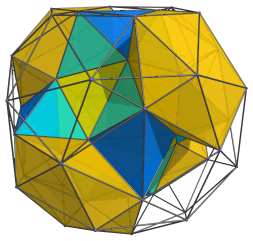The next image adds the remaining 4 icosahedra: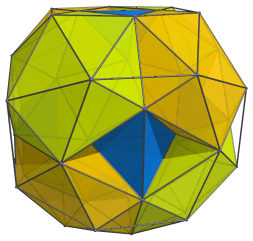The visible pairs of tetrahedral gaps are filled in by 12 more tetrahedra, half of which are shown next: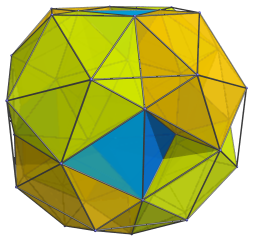The remaining gaps are filled with the remaining 6 tetrahedra in the next image: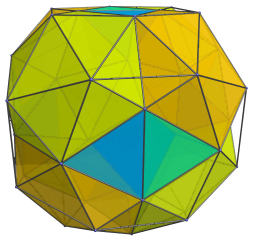Finally, the flattened tetrahedral gaps straddling each adjacent pair of icosahedra are filled in by another 12 tetrahedra: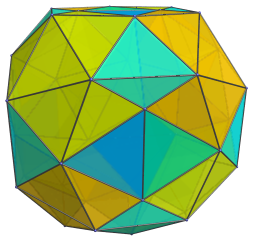### The Equator

We have now reached the “equator” of the snub 24-cell. There are 6 icosahedra that lie on the equator: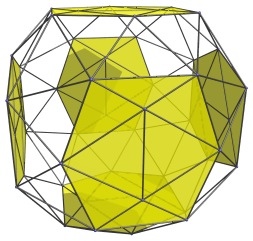For clarity, we omit the cells that we've seen in the northern hemisphere.

These icosahedral cells appear flattened into hexagons, because they are seen at a 90° angle. In reality, they are perfectly regular icosahedra.

Surrounding the hexagonal images of these icosahedra are rings of triangles; 24 of these triangles are the images of 24 tetrahedra which share a face with one of these icosahedra: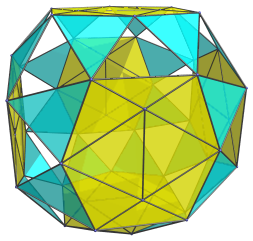Note that the shared edge between the triangular images of these tetrahedra and the hexagonal images of the icosahedra is actually a triangular face. It has been foreshortened into an edge because it is seen at a 90° angle.

Twelve of the remaining gaps are images of the second class of tetrahedra, which do not share a face with the icosahedra, but only with other tetrahedra. These are shown next: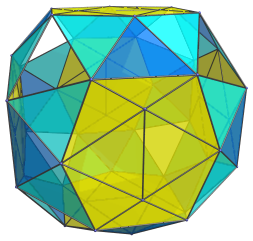These are all the cells that lie on the equator of the snub 24-cell. Past this point, the cells in the southern hemisphere perfectly mirror the arrangement of cells in the northern hemisphere.

The 8 remaining triangular gaps on the equator are not the projection images of any cell; they are the triangular faces where the 8 icosahedra in the northern hemisphere touch the corresponding icosahedra in the southern hemisphere.

### Summary

In summary, in the northern hemisphere there are 1+8=9 icosahedral cells, 12+12+12=36 tetrahedral cells of the first group, and 6 tetrahedral cells of the second group. The equator has 6 icosahedra cells, 24 tetrahedra of the first group, and 12 tetrahedra of the second group. The southern hemisphere, mirroring the northern hemisphere, has 9 icosahedral cells, 36 tetrahedra of the first group, and 6 tetrahedra of the second group. In total, there are 9+6+9=24 icosahedra, 36+24+36=96 tetrahedra of the first group, and 6+12+6=24 tetrahedra of the second group, for a total of 120 tetrahedra.

## Perspective Projection

The following image shows the perspective projection of the snub 24-cell into 3D, this time centered on one of the tetrahedra of the second group instead of an icosahedron.The nearest tetrahedron is shown in blue, at the center of the image. The pairs of red, green, and cyan cells are the 4 icosahedra sharing an edge with this tetrahedron. The remaining cells are colored in transparent yellow. As usual, for clarity we omit cells that lie on the far side of the snub 24-cell.

## Geometric Properties

### Construction

Just as the icosahedron can be derived from the octahedron by dividing the octahedron's edges in the golden ratio and truncating it at those points, so the snub 24-cell can be derived from the 24-cell by dividing its edges in the golden ratio and truncating it. The edges of the 24-cell, of course, need to be coherently indexed, that is, each edge needs to be assigned a direction such that the edges of every triangle form a circuit in a consistent direction. Once such an indexing is obtained, each edge is divided in the golden ratio according to its assigned direction, and the 24-cell is truncated at the points of division.

Such a truncation transforms the 24 octahedral cells of the 24-cell into the 24 icosahedral cells of the snub 24-cell. The truncated vertices become 24 tetrahedral cells corresponding to the cells of the dual 24-cell; these are the tetrahedra of the second group as described above. The remaining gaps in between, corresponding to the 24-cell's 96 edges, are filled in by another 96 tetrahedra, which are the tetrahedra of the first group.

### Relation to the 600-cell

The snub 24-cell can be used as an intermediate in constructing the 600-cell from the 24-cell. Shallow pyramids can be erected on its icosahedral cells, each consisting of an icosahedral base and 20 tetrahedra. If the apices of the pyramids lie at the same radius as the snub 24-cell's vertices, the resulting polychoron is the 600-cell. Conversely, another way to construct the snub 24-cell is to remove from the 600-cell the 24 vertices of an inscribed 24-cell and take the convex hull of the remaining vertices. This is equivalent to removing 24 icosahedral pyramids from the 600-cell.

### Coordinates

The Cartesian coordinates of the origin-centered snub 24-cell with edge-length 2 are all even permutations of coordinates and changes of sign of:

• (0, 1, φ, φ2)

where φ is the Golden Ratio, (1+√5)/2.

Last updated 17 Jun 2019.